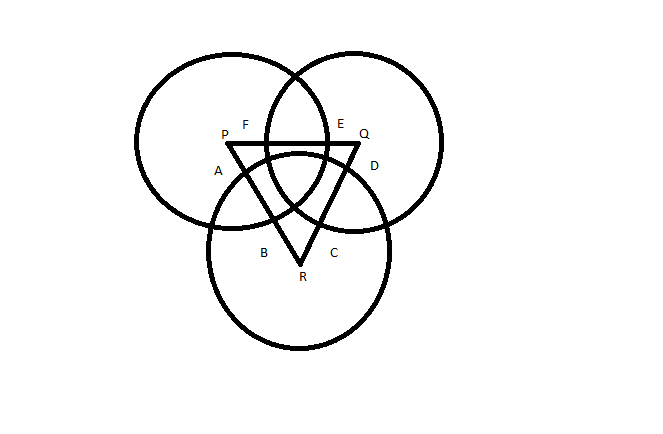Question 46

# Three circles, each of radius 20, have centres at P, Q and R. Further, AB = 5, CD = 10 and EF = 12. What is the perimeter of ΔPQR ?Solution

As radius of circle is 20, lengths of PR, QR and PQ will be (15+5+15) , (10+10+10) , (8+12+8) respectively.
So perimeter will be = 28 + 30 + 35 = 93

• All Quant CAT Formulas and shortcuts PDF
• 30+ CAT previous papers with solutions PDF

##### DKP Dev

3 years ago

I don't understand

##### s R

3 years ago

Bro consider the line PR .Now length of PB =20 and we know AB = 5 then ,PA =PB-AB =20-5=15 similarly PB will be 15 so overall length PR =PA+AB+BR=15+5+15=35

OR